Courses

# Chapter Notes - Visualizing Solid Shapes Class 7 Notes | EduRev

## Mathematics (Maths) Class 7

Created by: Praveen Kumar

## Class 7 : Chapter Notes - Visualizing Solid Shapes Class 7 Notes | EduRev

The document Chapter Notes - Visualizing Solid Shapes Class 7 Notes | EduRev is a part of the Class 7 Course Mathematics (Maths) Class 7.
All you need of Class 7 at this link: Class 7

15. Visualizing Solid Shapes

Introduction to Solid Shapes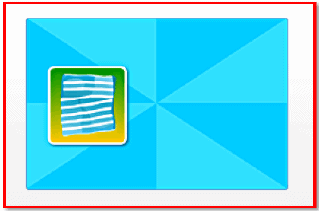All two-dimensional figures have only length and breadth.

All two-dimensional figures have only length and breadth.

For example, paper has only length and breadth, and hence, it is classified as a plane or two-dimensional figure.

Three-dimensional solid shapes have length, breadth and height.

For example, a biscuit tin is in three-dimensional shape.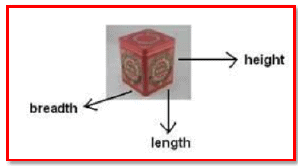Faces:

The flat surfaces that form the skin of solids are called faces.

Edges:

The line segments that form the skeleton of solids are called edges.

Page 60

Vertices:

The points where three edges meet are called vertices.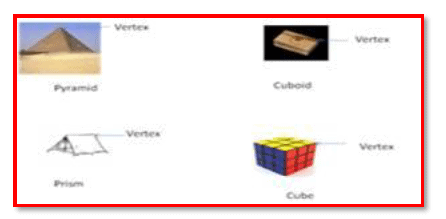The table shows the number of the faces, edges and vertices of some shapes.

 Face (F) Edge(E) Vertex (V)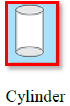3 2 0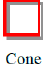2 1 1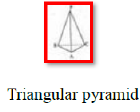4 6 4

The net of a three-dimensional solid is a two-dimensional skeleton outline, which, when folded, results in the three-dimensional shape.

Net solid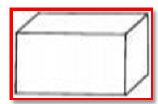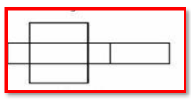Page 61

Solid shapes can be drawn on a flat surface, which is known as the two-dimensional representation of a three-dimensional solid.

Sketches of solids are of two types: oblique and isometric.

Oblique sketches are drawn on squared paper. They do not have exact dimensions, but still convey all the significant aspects of the appearance of a solid.

Isometric sketches are drawn on dotted or isometric sheets and have the exact measurements of solids.

The two-dimensional surface on which we draw an image is usually flat. So, when we try drawing a solid shape on a paper or a board, the image appears a little distorted. However, this is just an optical illusion. Look at the picture of the cube. Not all the lines forming the cube are of equal length. Also, we are unable to see all the faces of the cube. In spite of this illusion, we can make out that the image is of a cube. Such skeletons of solids are called oblique sketches. You can draw them using a squared paper. The first step is to draw the front face of the cube. Then draw the opposite face of the cube. This face should also be of the same size as that of the first square. Use the number of squares as a reference for maintaining the size. Now, join the corresponding corners of the squares. And finally, draw the edges that cannot be seen, with dotted lines. You can see that the front face and the opposite face of the cube are of the same size. Also, the edges appear equal, though we do not draw them of the same length.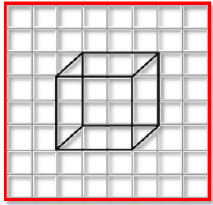All of us must have solved puzzles on isometric sheets at some point of time. An isometric sheet divides a screen into small equilateral triangles made of dots. Using this sheet, we can draw sketches with measurements that agree with that of a given solid. Let's try to draw the sketch of a cuboid of length three units, breadth two units and height two units. First, draw a rectangle of length three

Page 62

units and breadth two units. Then draw four parallel line segments, each of length two units, starting from the four corners of the rectangle. Finally, connect the matching corners with appropriate line segments. The isometric sketch of the cuboid is ready. Observe that the measurements are of the exact size, which is not the case with oblique sketches.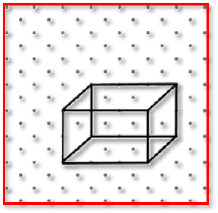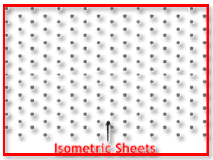Viewing the different section of Solids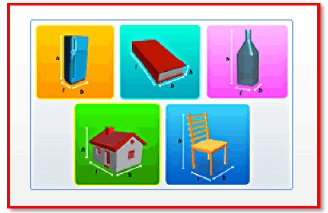Visualizing solid shapes is a very useful skill.

Visualizing solid shapes is a very useful skill. We can see the hidden parts of a solid shape. For example, when a cuboid with a square face is cut vertically, then each face is a square. The face is a cross section of the cuboid.

Page 63

Three-dimensional objects or solids have length, breadth and height, and look different from different points of view. Sections of a solid can be viewed in a number of ways. A solid can be viewed from different angles. Viewing a solid from the front, side and top are the three most common ways of viewing it.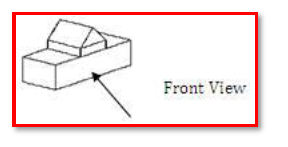Cutting or slicing a solid will result in its cross-section, which is also one way of viewing it.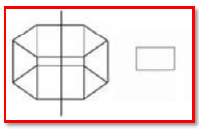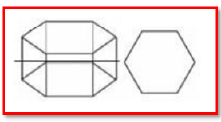Observing the two-dimensional shadow of a three-dimensional solid is another method of viewing a solid. Shadows of solids are of different sizes depending on the position of the solid and the position of the source of light.

,

,

,

,

,

,

,

,

,

,

,

,

,

,

,

,

,

,

,

,

,

;